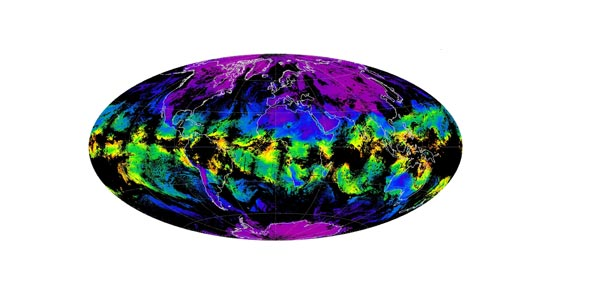# Earth's Atmosphere - Chapter 1

19 Questions | Total Attempts: 526SettingsThe earth is the only planet in the spectrum that boasts of its air combination. The atmosphere is made up of different layers of air space, all of which have different characteristics. How knowledgeable are you on this? Find out.

Related Topics
• 1.
Where is Earth's atmosphere most dense?
• A.

At its bottom

• B.

At its top

• C.

On a mountaintop

• D.

At the height jets fly

• 2.
Earth's atmosphere is mostly
• A.

Oxygen and hydrogen

• B.

Nitrogen and water vapor

• C.

Carbon cycle

• D.

Nitrogen and oxygen

• 3.
Plants take carbon dioxide from the air as part of the
• A.

Water cycle

• B.

Nitrogen cycle

• C.

Carbon cycle

• D.

Volcanic cycle

• 4.
Which of these statements is true?
• A.

Water is exchanged with nitrogen in a natural cycle

• B.

The water cycle involves only the liquid form of water

• C.

Ongoing life processes are part of the nitrogen cycle

• D.

Water is exchanged with carbon in a natural cycle

• 5.
• A.

A rainstorm in a mountainous area

• B.

The eruption of a very large volcano

• C.

The release of oxygen by plants

• D.

The decay of animals after they die

• 6.
the transfer of energy from place to place in the form of certain types of waves is _______________
• A.

Absorbs

• B.

• C.

Conduction

• D.

Density

• 7.
the ground is heated by _____________ from the sun
• A.

Absorbs

• B.

Density

• C.

• D.

Reflects

• 8.
when warm air moves upward, energy is moved by ___________
• A.

Convection

• B.

Conduction

• C.

• D.

Temperature

• 9.
ozone gas _______________ solar energy and heats the atmosphere
• A.

Absorbs

• B.

Conduction

• C.

Density

• D.

Reflects

• 10.
_________________ is the transfer of energy of energy by direct contact
• A.

• B.

Convection

• C.

Conduction

• D.

Absorbs

• 11.
what happens to most visible light when it enters the atmosphere?
• A.

It is reflected

• B.

It passed through

• C.

It is emitted

• D.

It is absorbed

• 12.
which type of radiation waves have more energy than waves of radiation that you can see?
• A.

Ultraviolet

• B.

Infrared

• C.

Visible

• D.

Greenhouse

• 13.
which of the following is true?
• A.

Greenhouse gases absorb and give off ultraviolet radiation

• B.

Greenhouse gases absorb and give off infrared radiation

• C.

Greenhouse gases absorb solar radiation and keep Earth's surface from heating up

• D.

Greenhouse gases reflect radiation from Earth's atmosphere to outer space

• 14.
ozone is a molecule that is
• A.

Made of three atoms of the element oxygen

• B.

Made of one carbon dioxide and one water molecule

• C.

The main ingredient in the stratosphere

• D.

Mainly responsible for the greenhouse effect

• 15.
human activity is the only source of pollution
• A.

True

• B.

False

• 16.
air pollutants affect only living things
• A.

True

• B.

False

• 17.
particulates are gaseous pollutants mixed with air
• A.

True

• B.

False

• 18.
the burning of fossil fuels produces both air pollution and greenhouse gases
• A.

True

• B.

False

• 19.
a combination of pollutants formed in the presence of sunlight is just one of the two types of smog
• A.

True

• B.

False